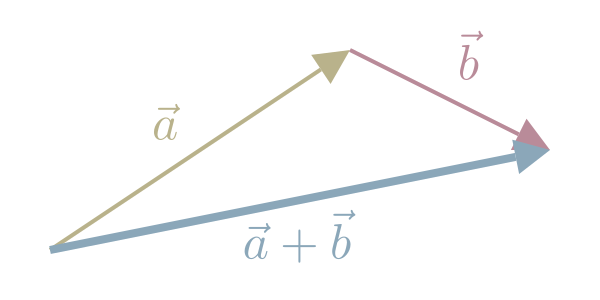$\vec{a} + \vec{b} = \begin{bmatrix} a_1 \\ a_2 \end{bmatrix} + \begin{bmatrix} b_1 \\ b_2 \end{bmatrix} = \begin{bmatrix} a_1 + b_1 \\ a_2 + b_2 \end{bmatrix}$

Vector addition requires both vectors to have equal dimensions.

We can visualize Vector addition as follows:

1. draw the 1st Vector
2. draw the 2nd Vector starting from the tip of the 1st Vector
3. Draw a line from the tail of the 1st Vector to the tip of the 2nd.3Blue1Brown (2016)

#### References

3Blue1Brown. Vectors | Chapter 1, Essence of linear algebra. August 2016. URL: https://www.youtube.com/watch?v=fNk_zzaMoSs.